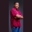Related Tags

scala
array
largest
communitycreator

# How to find the largest number in an array in ScalaTheodore Kelechukwu Onyejiaku

## Overview

We can easily use the while loop and integer variables to find the largest number of elements of an array in Scala.

### Syntax

In Scala, the syntax for a while loop is as follows:

while(condition){
// code body
}
Syntax for the while loop

### Parameters

condition: This is a conditional statement. If it returns true, the code body will execute. Else, the code body will not execute

### Code

Here's an example of finding the largest element in an array.

object Main extends App {
// create default variables
var OurArray = Array(1, 5, 4, 2, 3)
var counter = 0
var largest = 0

// create a while loop
while(counter < OurArray.size) // if less than size of array
{
if(largest < OurArray(counter))
// make current element largest
largest = OurArray(counter)

// increase counter after every loop
counter = counter + 1
}

// after the loop print highest value
println("The highest value is "+largest)
}
Find the largest number of an array in Scala

### Explanation

• Lines 3–5: Create an array and two variables. Use counter in the loop to keep count of every loop. Use the largest to obtain the largest number after the loop.
• Line 8: Creates a while loop. The code body shall run if the counter is less than the array size that is 5 as per the condition.
• Line 10: We use an if condition to check if the previous largest value is less than the array element. If so, then the largest becomes that array element. This is how the code will run until we obtain the largest element.
• Line 15: Increase the counter for every loop. This is so that we can access each element of the array as, OurArray(counter).
• Line 19: Prints the largest element.

RELATED TAGS

scala
array
largest
communitycreator

CONTRIBUTORTheodore Kelechukwu Onyejiaku
RELATED COURSES

View all Courses

Keep Exploring

Learn in-demand tech skills in half the time